Get instant live expert help with Excel or Google Sheets“My Excelchat expert helped me in less than 20 minutes, saving me what would have been 5 hours of work!”

#### Post your problem and you’ll get Expert help in seconds.

Your message must be at least 40 characters
Our professional Expert are available now. Your privacy is guaranteed.

# Get days, months, and years between dates

Duration between two dates can be determined by using the DATEDIF function.  This step by step tutorial will assist all levels of Excel users in calculating the difference between dates in days, months and years.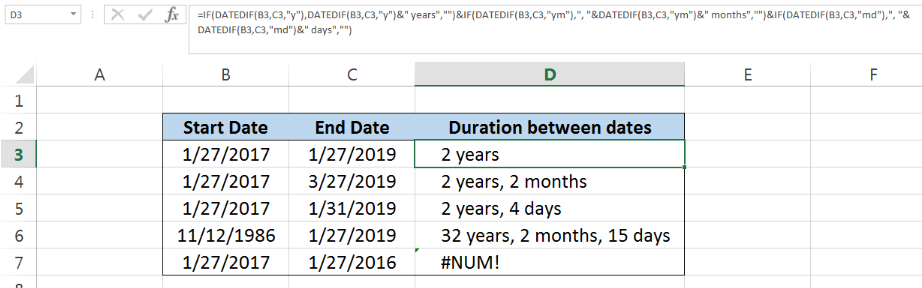Figure 1. Final result: Get days, months, and years between dates

Final formula:

`=IF(DATEDIF(B3,C3,"y"),DATEDIF(B3,C3,"y")&" years","")&IF(DATEDIF(B3,C3,"ym"),", "&DATEDIF(B3,C3,"ym")&" months","")&IF(DATEDIF(B3,C3,"md"),", "&DATEDIF(B3,C3,"md")&" days","")`

## Syntax of IF Function

IF function evaluates a given logical test and returns a TRUE or a FALSE

`=IF(logical_test, [value_if_true], [value_if_false])`

• The arguments “value_if_true” and “value_if_false” are optional.  If left blank, the function will return TRUE if the logical test is met, and FALSE if otherwise.

## Syntax of DATEDIF Function

DATEDIF function returns the difference between two dates in number of years, months or days;  it returns a #NUM! error when start_date is greater than the end_date

`=DATEDIF(start_date,end_date,unit)`

• start_date  – a date representing the start date
• end_date  – a date representing the end date
• Dates may be entered as serial numbers, text strings in quotation marks, or cell reference
• unit  – represents the type of information we want to obtain; we can refer to the table below: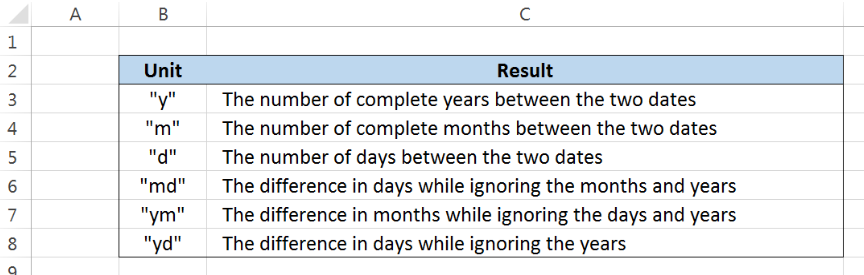Figure 2.  Unit options and corresponding results for DATEDIF function

## Setting up the Data

Our table has three columns: Start Date (column B), End Date (column C) and Duration between dates (column D).  We want to determine the duration between the start date and end date in number of years, months and days. The results will be recorded in column D.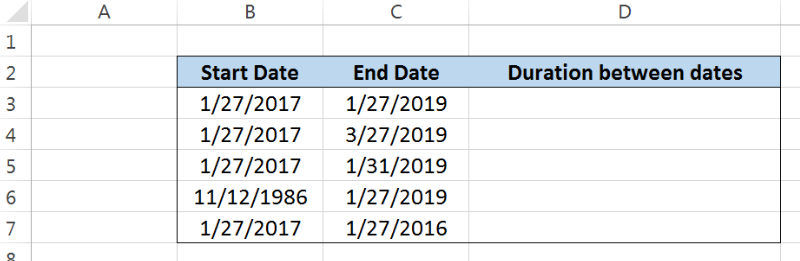Figure 3. Sample data to get days, months, and years between dates

## Duration between dates in days, months and years

In order to determine the difference between two dates in number of days, months and years, we use the DATEDIF function.  Let us follow these steps:

Step 1. Select cell D3

Step 2. Enter the formula:

`=DATEDIF(B3,C3,"y")&" years"&", "&DATEDIF(B3,C3,"ym")&" months"&", "&DATEDIF(B3,C3,"md")&" days"`

Step 3. Press Enter

Step 4:  Copy the formula in cell D3 to cells D4:D7 by clicking the “+” icon at the bottom-right corner of cell D3 and dragging it down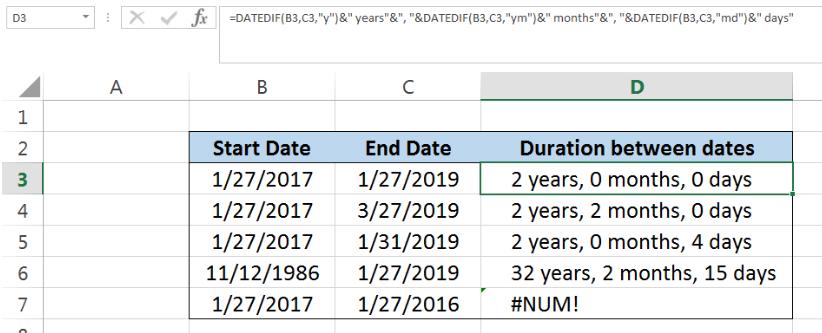Figure 4.  Entering the DATEDIF function to get days, months, and years between dates

Our formula consists of three DATEDIF functions where the number of years, months and days are calculated separately.  The unit arguments used for number of years, months and days are “y”, “ym” and “md”, respectively.

Each DATEDIF function calculates the difference between the dates in the specified unit, then links the appropriate text string for “years”, “months” and “days”.  The ampersand “&” is used to link text strings together.

We can break down the formula into three components:

For years: `DATEDIF(B3,C3,"y")&" years"`

For months: `&", "&DATEDIF(B3,C3,"ym")&" months"`

For days: `&", "&DATEDIF(B3,C3,"md")&" days"`

As a result, the values in D3:D6 represent the duration between the two dates in columns B and C in number of years, months and days.  For cell D7, our formula returns a #NUM! error because the start date “1/27/2017” is greater than the end date “1/27/2016”.

## Duration between dates without zero values

Note that the results in column D in the previous example include some zero values.  We want to determine the difference between two dates without having to show the zero values.  We can do this by using a formula that combines the IF and DATEDIF functions. Let us follow these steps:

Step 1. Select cell D3

Step 2. Enter the formula:

`=IF(DATEDIF(B3,C3,"y"),DATEDIF(B3,C3,"y")&" years","")&IF(DATEDIF(B3,C3,"ym"),", "&DATEDIF(B3,C3,"ym")&" months","")&IF(DATEDIF(B3,C3,"md"),", "&DATEDIF(B3,C3,"md")&" days","")`

Step 3. Press Enter

Step 4:  Copy the formula in cell D3 to cells D4:D7 by clicking the “+” icon at the bottom-right corner of cell D3 and dragging it down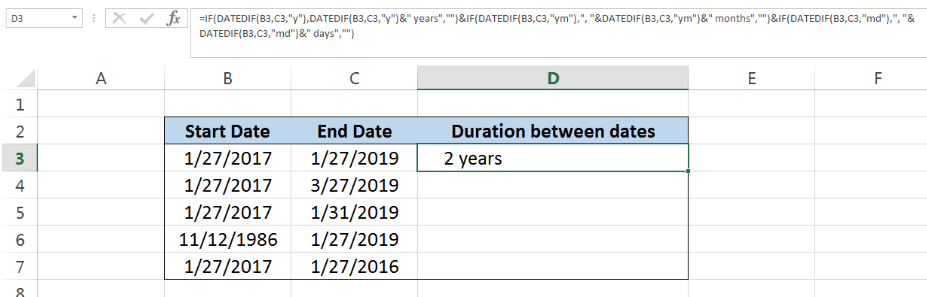Figure 5.  Entering the IF and DATEDIF functions to show only non-zero values

This time, our DATEDIF formula is embedded in an IF formula.  The IF function evaluates non-zero results as true. Hence, if the DATEDIF function returns a value other than zero, the IF function will return the result of the DATEDIF function; otherwise it returns an empty string “”.

Below table shows the simplified results of our formula, where zero values are not displayed.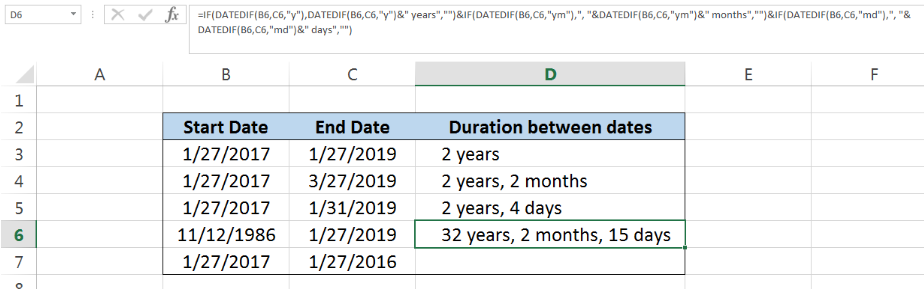Figure 6.  IF and DATEDIF showing the difference in dates without zero values

Most of the time, the problem you will need to solve will be more complex than a simple application of a formula or function. If you want to save hours of research and frustration, try our live Excelchat service! Our Excel Experts are available 24/7 to answer any Excel question you may have. We guarantee a connection within 30 seconds and a customized solution within 20 minutes.

Solution examplesCould you help me by explaining the IF function?
Solved by G. L. in 24 minsI have a question about Conditional Formatting. =IF('Sheet1'!A1="X",IF('Sheet1'!B1="Y", TRUE, FALSE),FALSE) the set the cell background to red. I would like to make it so that if Sheet1'!B1="Z" I set the back to blue. How can I achieve this.
Solved by B. F. in 39 minsI need to find out if the 2018 yearly sales goals were met if the yearly sales were \$25,000 or more using an IF logical function and to set the formula to return a value of YES if met, and NO if not
Solved by A. A. in 18 minsI filtered data in column A (it is labeled ID #) of a data set. On my next worksheet, column A is also the ID #, but it is a different data set. I want to filter the ID #'s the same for the two sheets, but how do I do that being that I am working with two different sets of data?
Solved by V. H. in 22 minsi want to create a very simple if function in a cell which is, if one cell is less than 1250, then the automatic value in the current cell is 250, dead easy, for someone who knows! Thank you.
Solved by A. H. in 14 mins